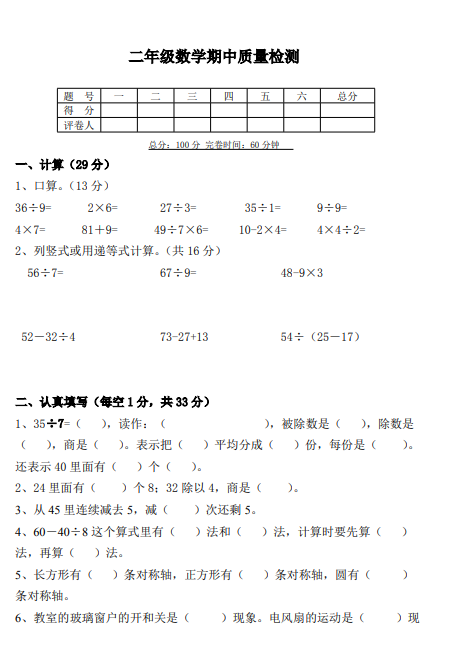1、口算。（13 分）
36÷9= 2×6= 27÷3= 35÷1= 9÷9=
4×7= 81＋9= 49÷7×6= 10-2×4= 4×4÷2=
2、列竖式或用递等式计算。（共 16 分）
56÷7= 67÷9= 48-9×3
52－32÷4 73-27+13 54÷（25－17）##### 评论信息